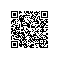# iBATIS In Action：执行非查询语句（二）

• iBATIS API的更多内容
• 插入数据
• 更新和删除数据
• 使用存储过程

5.3 更新和删除数据

Insert方法返回的是object类型的值，而UpdateDelete方法则返回int类型的值，该值指示了更新或删除语句所影响的记录数。

iBATIS框架允许使用单条语句操作一条或多条记录。这是它与大多数ORM工具不同的地方之一，后者一般只能修改单条记录。

5.3.1 并发更新处理

iBATIS目前尚未实现的一个功能是锁定记录以管理对相同数据的并发修改。有几种技术可用来处理并发更新，如在数据行上使用时间戳或者版本号。比如，有如下一个account表，定义为：

CREATE TABLE account (
accountid serial
NOT NULL,
varchar(10),
passwd
varchar(10),
firstname
varchar(30),
lastname
varchar(30),
varchar(30),
varchar(30),
city
varchar(30),
state
varchar(5),
postalcode
varchar(10),
country
varchar(5),
version int8,
CONSTRAINT account_pkey PRIMARY KEY (accountid)
)

version列，在update语句的where子句使用accountIdversion两个字段。在执行更新操作时，如果要更新的记录未被其它用户修改，那么更新会得以成功地执行，因为版本号未被改变，映射语句也可返回期望的记录数。如果返回的是0，而且未抛出任何异常，那么您就该知道，其他人已经修改了记录。下一步如何处理就由您来决定了。

5.3.2 更新和删除子项记录

public void SaveOrder(Order order)
{

if (null == order.OrderId)
{
sqlMapper.Insert(
"Order.insert", order);
}

else
{
sqlMapper.Update(
"Order.update", order);
}

sqlMapper.Delete(
"Order.deleteDetails", order);

for(int i = 0; i < order.OrderItems.Count; i++
{
OrderItem oi
= order.OrderItems[i];
sqlMapper.Insert(
"OrderItem.insert", oi);
}
}

5.4 执行批量更新

5.5 使用存储过程

5.5.1 三思而行

try
{
sqlMapper.BeginTransaction();
sqlMapper.QueryForObject(
"Account.insertAndReturn", a);
sqlMapper.CommitTransaction();
}

catch(Exception)
{
sqlMapper.RollBackTransaction();
}

7章，因此要获得更多信息，去那里看看吧。

5.5.2 INOUTINOUT参数

iBATIS中使用存储过程时，IN参数用法很简单。将参数传给存储过程跟传给映射语句是一样的。这里是一个简单的存储过程，它接受两个IN参数并返回一个值：

CREATE OR REPLACE FUNCTION max_in_example
(a float4, b float4)
RETURNS float4 AS
BODYBODY
BEGIN
if (a > b) then
return a;
else
return b;
end if;
END;
BODYBODY
LANGUAGE
'plpgsql' VOLATILE;

<parameterMap id="pm_in_example" class="hashtable">

<parameter property="a" column="a" />

<parameter property="b" column="b" />
</parameterMap>

<procedure id="in_example" parameterMap="pm_in_example" resultClass="int" >
max_in_example
</procedure>

Hashtable condition = new Hashtable();
"a"7);
"b"5);
return sqlMapper.QueryForObject<int>("Account.in_example", condition);

INOUT参数是指那些可以传给存储过程，可被存储过程修改的参数，就像在下面的例子中，存储过程接受两个数字，交换它们的值。看存储过程的代码（使用MS SQLT-SQL）：

CREATE PROCEDURE Swap

@a INT OUTPUT,

@b INT OUTPUT
AS

DECLARE @temp INT

SET @temp = @a

SET @a = @b

SET @b = @temp

<parameterMap id="para_swap_numbers" class="hashtable">

<parameter property="a" column="a" direction="InputOutput" />

<parameter property="b" column="b" direction="InputOutput" />
</parameterMap>

<procedure id="swap_numbers" parameterMap="para_swap_numbers">
Swap
</procedure>

Hashtable condition = new Hashtable();
"a"7);
"b"5);
sqlMapper.Insert(
"swap_numbers", condition);

OUT参数较为少用。它类似于结果（就像resultMap中的那样），却像参数那样传入。传入的值会被忽略，然后在存储过程中被返回值替换掉。OUT参数可返回任意的单个值（见我们的下个例子）。 下面例子中的存储过程很简单，使用两个IN参数和一个OUT参数（MS SQLT-SQL）:

CREATE PROCEDURE Max

@a INT,

@b INT,

@c INT OUTPUT
AS

IF @a > @b

SET @c = @a

ELSE

SET @c = @b

<procedure id="max_number" parameterMap="para_max_number">
Max
</procedure>

<parameterMap id="para_max_number" class="hashtable">

<parameter property="a" column="a" direction="Input" />

<parameter property="b" column="b" direction="Input" />

<parameter property="c" column="c" direction="Output" dbType="int" />
</parameterMap>

Hashtable condition = new Hashtable();
"a", a);
"b", b);

ExecuteInsert(
"max_number", condition);
//Convert.ToInt32(condition["c"]) is the maximum.

5.6 小结使用钉钉扫一扫加入圈子
+ 订阅